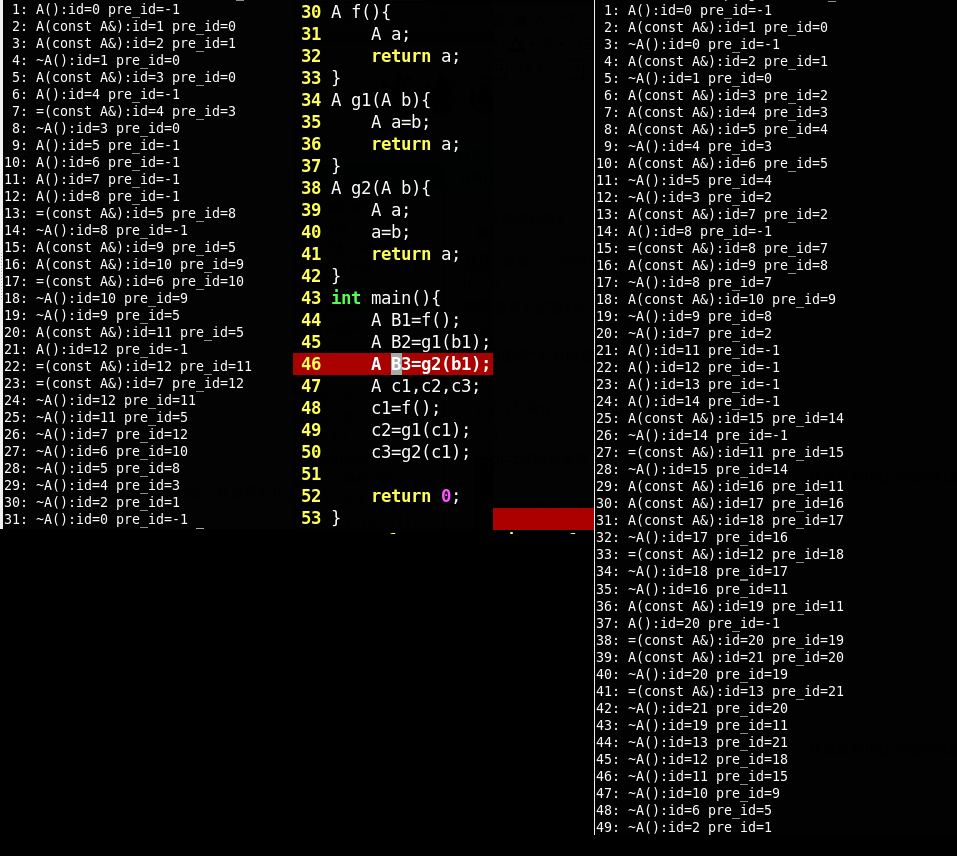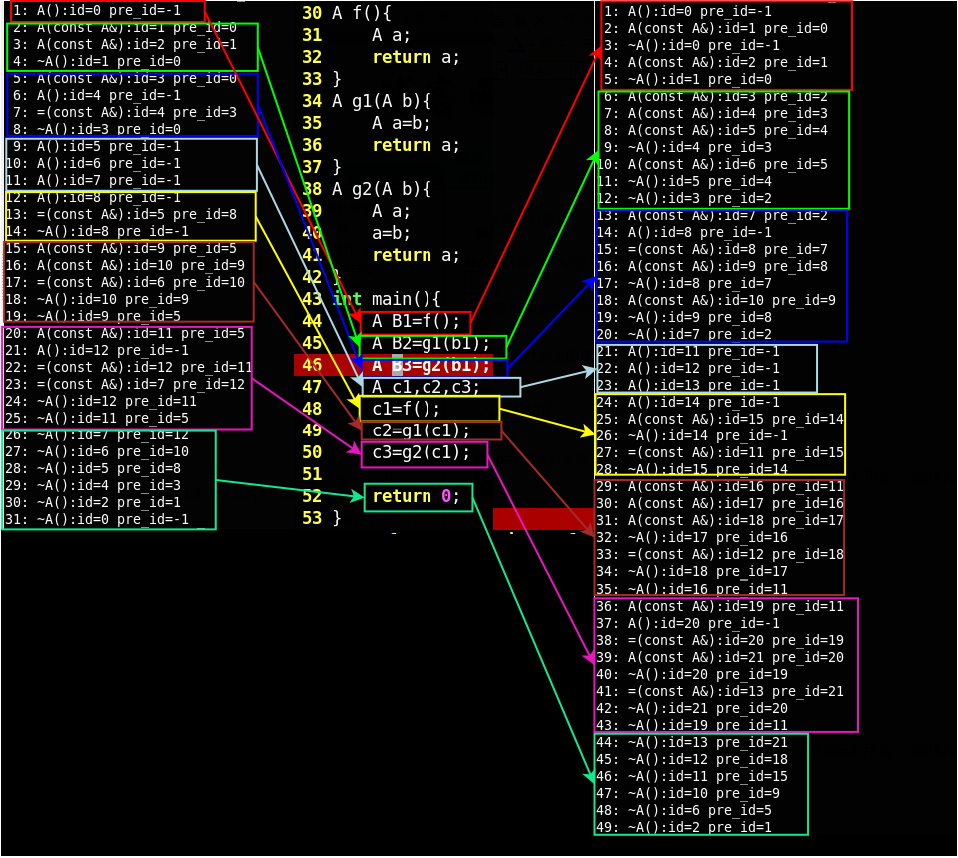# C++中返回对象的情形及RVO

g++ -o rvo_test rvo_test.cc -fno-elide-constructors

#include <iostream>
#include <iomanip>
using namespace std;
int num=1;
class A{
public:
A(){
id=count++;
pre_id=-1;
cout<<setw(2)<<num++<<": A():id="<<id<<" pre_id="<<pre_id<<endl;
}
A(const A& a){
id=count++;
pre_id=a.id;
cout<<setw(2)<<num++<<": A(const A&):id="<<id<<" pre_id="<<pre_id<<endl;
}
~A(){
cout<<setw(2)<<num++<<": ~A():id="<<id<<" pre_id="<<pre_id<<endl;
}
A& operator=(const A& a){
pre_id=a.id;
cout<<setw(2)<<num++<<": =(const A&):id="<<id<<" pre_id="<<pre_id<<endl;
}
private:
static int count;
int id;
int pre_id;
};
int A::count=0;
A f(){
A a;
return a;
}
A g1(A b){
A a=b;
return a;
}
A g2(A b){
A a;
a=b;
return a;
}
int main(){
A B1=f();
A B2=g1(b1);
A B3=g2(b1);
A c1,c2,c3;
c1=f();
c2=g1(c1);
c3=g2(c1);

return 0;
}

为了便于区分每一个对象，采用变量id来记录对象的标号。实现方式是采用了一个静态变量count来记录生成类的个数。对右面的运行结果进行解析如下：

（1）. 对于 A B1=f();

A f(){
A a;
return a;
}

1: A():id=0 pre_id=-1

2: A(const A&):id=1 pre_id=0

3: ~A():id=0 pre_id=-1

A B1=f();

4: A(const A&):id=2 pre_id=1

5: ~A():id=1 pre_id=0

（2）.对于A B2=g1(B1);

A g1(A b){
A a=b;
return a;
}

6: A(const A&):id=3 pre_id=2

A a=b;

7: A(const A&):id=4 pre_id=3

8: A(const A&):id=5 pre_id=4

9: ~A():id=4 pre_id=3

10: A(const A&):id=6 pre_id=5

11: ~A():id=5 pre_id=4

12: ~A():id=3 pre_id=2

（3).对于A B3=g2(B1);

A g2(A b){
A a;
a=b;
return a;
}
g2与g1的不同之处在于g2中是进行了赋值，而不是直接调用复制构造函数来生成局部变量。

13: A(const A&):id=7 pre_id=2

14: A():id=8 pre_id=-1

15: =(const A&):id=8 pre_id=7

16: A(const A&):id=9 pre_id=8

17: ~A():id=8 pre_id=7

18: A(const A&):id=10 pre_id=9

19: ~A():id=9 pre_id=8

20: ~A():id=7 pre_id=2

（4）下面几句运行的方式和前3句类似，只是：先调用默认构造函数生成对象，然后在调用赋值操作符进行赋值。

A c1,c2,c3;
c1=f();
c2=g1(c1);
c3=g2(c1);

21: A():id=11 pre_id=-1
22: A():id=12 pre_id=-1
23: A():id=13 pre_id=-1

24: A():id=14 pre_id=-1
25: A(const A&):id=15 pre_id=14
26: ~A():id=14 pre_id=-1
27: =(const A&):id=11 pre_id=15
28: ~A():id=15 pre_id=14

29: A(const A&):id=16 pre_id=11
30: A(const A&):id=17 pre_id=16
31: A(const A&):id=18 pre_id=17
32: ~A():id=17 pre_id=16
33: =(const A&):id=12 pre_id=18
34: ~A():id=18 pre_id=17
35: ~A():id=16 pre_id=11

36: A(const A&):id=19 pre_id=11
37: A():id=20 pre_id=-1
38: =(const A&):id=20 pre_id=19
39: A(const A&):id=21 pre_id=20
40: ~A():id=20 pre_id=19
41: =(const A&):id=13 pre_id=21
42: ~A():id=21 pre_id=20
43: ~A():id=19 pre_id=11

44: ~A():id=13 pre_id=21//销毁对象c3（id=13）

45: ~A():id=12 pre_id=18//销毁对象c2（id=12）
46: ~A():id=11 pre_id=15//销毁对象c2（id=11）
47: ~A():id=10 pre_id=9//销毁对象B3（id=10）
48: ~A():id=6 pre_id=5//销毁对象B2（id=6）
49: ~A():id=2 pre_id=1////销毁对象B3（id=2）

(1)对于A B1=f();

A f(){
A a;
return a;
}

1: A():id=0 pre_id=-1

（2）对于A B2=g1(B1);

A g1(A b){
A a=b;
return a;

2: A(const A&):id=1 pre_id=0

3: A(const A&):id=2 pre_id=1
4: ~A():id=1 pre_id=0

（3）对于A B3=g2(B1);

A g2(A b){
A a;
a=b;
return a;
}

5: A(const A&):id=3 pre_id=0
6: A():id=4 pre_id=-1
7: =(const A&):id=4 pre_id=3
8: ~A():id=3 pre_id=0

（4）对于A c1,c2,c3;

9: A():id=5 pre_id=-1
10: A():id=6 pre_id=-1
11: A():id=7 pre_id=-1

（5）对于下面三句：

c1=f();
c2=g1(c1);
c3=g2(c1);

12: A():id=8 pre_id=-1//由于需要利用f的返回值进行赋值操作，所以在f中调用默认构造函数直接生成一个临时变量（相当于省略了局部变量a）id=8
13: =(const A&):id=5 pre_id=8//利用临时变量id=8对c1（id=5）进行赋值操作。
14: ~A():id=8 pre_id=-1//临时变量id=8完成任务，销毁
15: A(const A&):id=9 pre_id=5//利用c1（id=5）复制构造形式参数b（id=9）
16: A(const A&):id=10 pre_id=9//利用形式参数b（id=9）复制构造一个临时变量id=10（省略局部变量a）
17: =(const A&):id=6 pre_id=10//利用临时变量id=10对c2（id=6）进行赋值操作
18: ~A():id=10 pre_id=9//销毁临时变量id=10
19: ~A():id=9 pre_id=5//销毁形式参数b对应临时变量id=9
20: A(const A&):id=11 pre_id=5//利用c1（id=5）复制构造形式参数b（id=11）
21: A():id=12 pre_id=-1//因为在g2函数中进行了赋值操作，并且需要返回局部变量a，这儿是省略了构造局部变量a，直接构造一个临时变量id=12
22: =(const A&):id=12 pre_id=11//利用形式参数b（id=11）对临时变量id=12进行赋值。

23: =(const A&):id=7 pre_id=12//利用临时变量id=12对c3（id=7）进行赋值。
24: ~A():id=12 pre_id=11//临时变量id=12完成任务，销毁
25: ~A():id=11 pre_id=5//销毁形式参数b对应临时变量id=11

下面就是程序即将运行完毕，销毁main函数中的所有局部变量：

26: ~A():id=7 pre_id=12//销毁对象c3（id=7）
27: ~A():id=6 pre_id=10//销毁对象c2（id=6）
28: ~A():id=5 pre_id=8//销毁对象c1（id=5）
29: ~A():id=4 pre_id=3//销毁对象B3（id=4）
30: ~A():id=2 pre_id=1//销毁对象B2(id=2)
31: ~A():id=0 pre_id=-1//销毁对象B1（id=0）posted @ 2012-07-21 11:23  Mr.Rico  阅读(...)  评论(...编辑  收藏